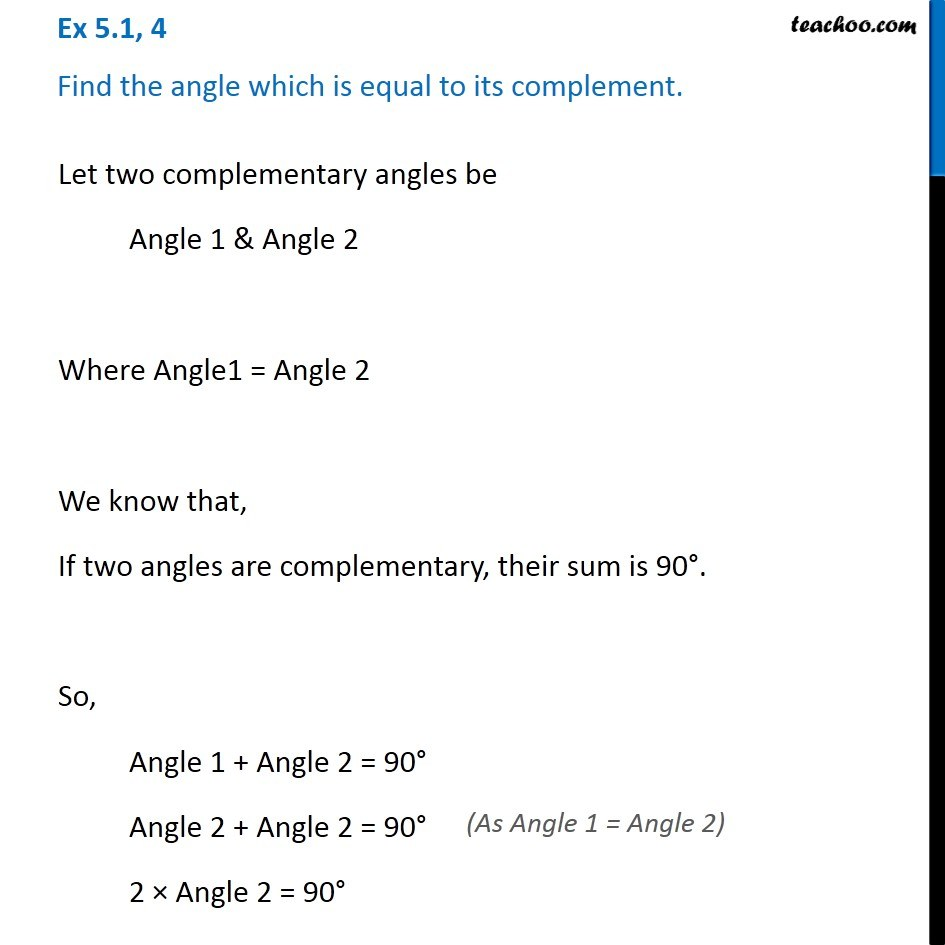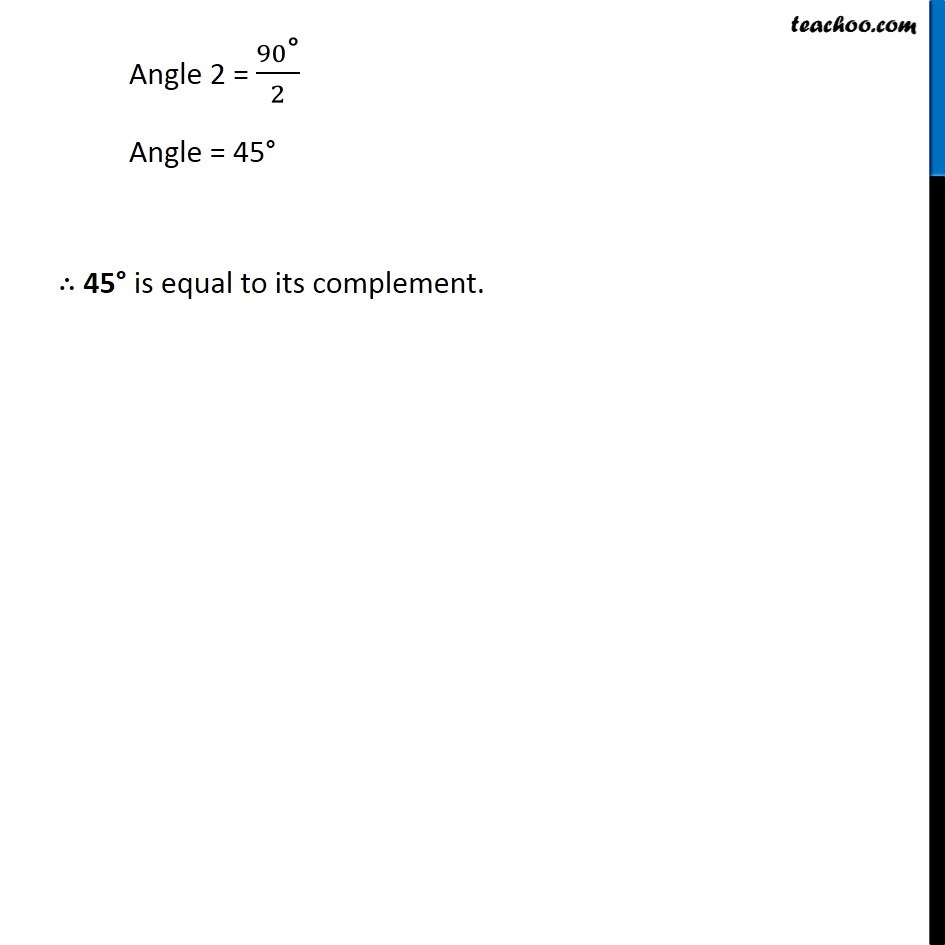Ex 5.1

Chapter 5 Class 7 Lines and Angles
Serial order wiseLearn in your speed, with individual attention - Teachoo Maths 1-on-1 Class

### Transcript

Ex 5.1, 4 Find the angle which is equal to its complement. Let two complementary angles be Angle 1 & Angle 2 Where Angle1 = Angle 2 We know that, If two angles are complementary, their sum is 90°. So, Angle 1 + Angle 2 = 90° Angle 2 + Angle 2 = 90° 2 × Angle 2 = 90° Angle 2 = 90"°" /2 Angle = 45° ∴ 45° is equal to its complement.